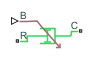# Variable Rotational Damper

Rotational damper with variable damping coefficient

•Libraries:
Simscape / Driveline / Couplings & Drives / Springs & Dampers

## Description

The Variable Rotational Damper block represents a rotational viscous damper with a variable damping coefficient. A physical signal input port provides the magnitude of the damping coefficient. The magnitude of the damping torque is equal to the product of the physical signal input and the relative angular velocity between the two rotational conserving ports, R and C. A minimum damping coefficient prevents nonphysical negative damping values.

The damping torque satisfies the following expression:

`$T=\left\{\begin{array}{cc}B\cdot \omega & B\ge {B}_{\mathrm{min}}\\ {B}_{\mathrm{min}}\cdot \omega & B<{B}_{\mathrm{min}}\end{array},$`

where

• T is the torque transmitted through damper.

• B is the viscous damping coefficient.

• ω is the relative angular velocity measured between the two rotational conserving ports according to $\omega ={\omega }_{R}-{\omega }_{C}$.

• ωR is the angular velocity of the rotational conserving port R.

• ωC is the angular velocity of the rotational conserving port C.

The block applies equal and opposite damping torques on the rotational conserving ports. The sign of the damping torque acting on port R is equal to the sign of the relative angular velocity. A positive relative angular velocity corresponds to a positive damping torque acting on port R, and a negative damping torque of equal magnitude acting on port C.

### Assumptions and Limitations

• The block represents only viscous damping.

• The value of the damping coefficient must be equal to or greater than zero.

## Ports

### Input

expand all

Physical signal input port associated with the variable damping coefficient.

### Conserving

expand all

Rotational mechanical conserving port associated with the base.

Rotational mechanical conserving port associated with the follower.

## Parameters

expand all

Minimum value allowed for the damping coefficient. The physical signal input saturates below this level.

## Version History

Introduced in R2013a